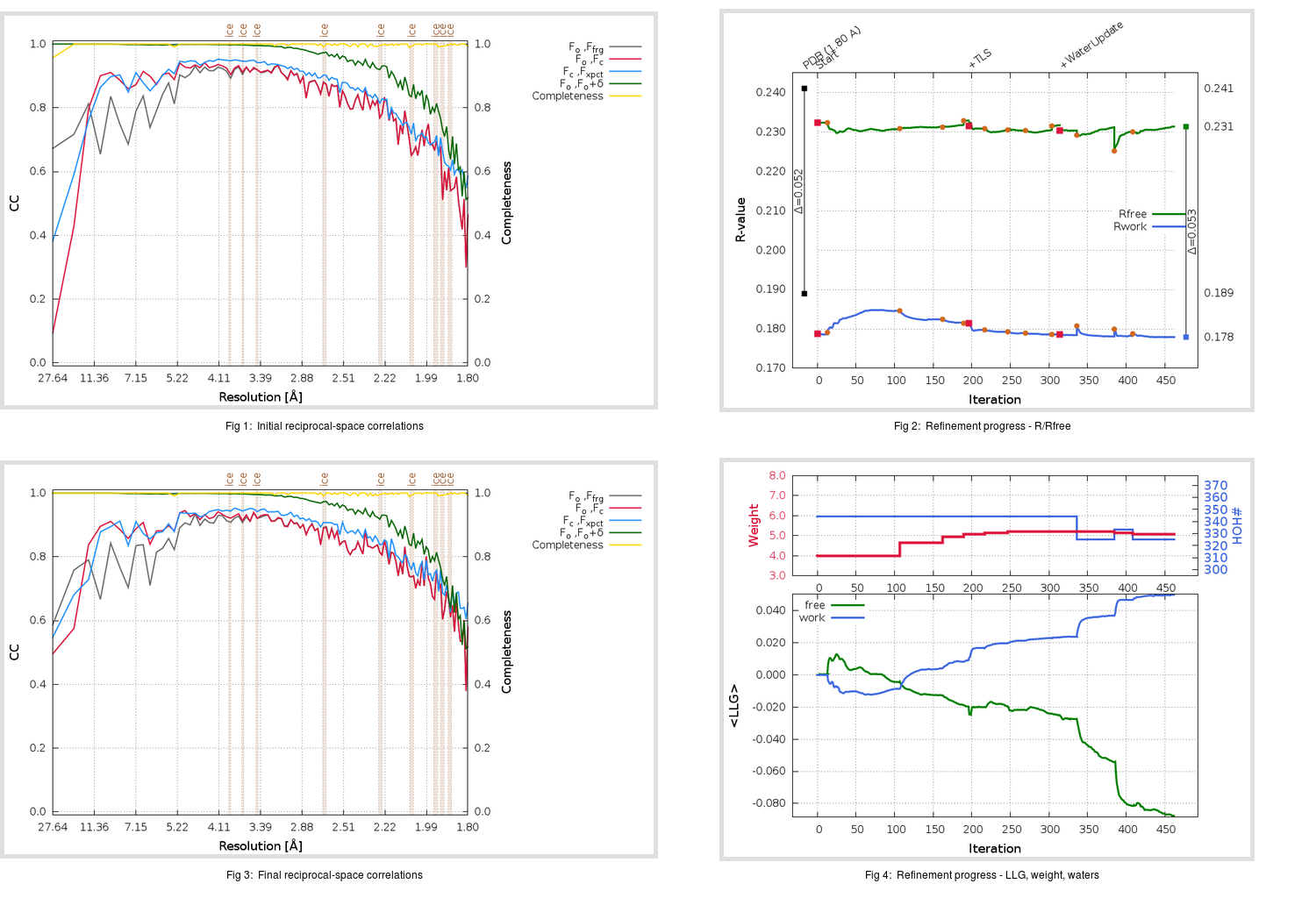Content:

## Deposited

` `
 Date deposited Date data collection Resolution R, Rfree 20200315 20200305 1.80 0.1860 0.2410

Molprobity (CCP4 7.0 version) summary:

```Ramachandran outliers =   0.33 %
favored =  97.68 %
Rotamer outliers      =   2.22 %
C-beta deviations     =     0
Clashscore            =   5.18
RMS(bonds)            =   0.0130
RMS(angles)           =   1.81
MolProbity score      =   1.61
Resolution            =   1.80
R-work                =   0.1860
R-free                =   0.2410
```

```Number of waters      =   344

<B> (all atoms) =   24.42 ( sd =    9.21 ) for       2787 non-hydrogen atoms
<B>   (protein) =   23.07 ( sd =    8.35 ) for       2416 non-hydrogen atoms
<B>     (water) =   33.62 ( sd =   10.06 ) for        344 non-hydrogen atoms
<B>    (others) =   30.25 ( sd =    9.89 ) for         27 non-hydrogen atoms

B min/max       (all non-hydrogen atoms) =   11.74 /   74.27
B min/max   (protein non-hydrogen atoms) =   12.25 /   74.27
B min/max     (water non-hydrogen atoms) =   11.74 /   70.57
B min/max     (other non-hydrogen atoms) =   22.07 /   45.24
```

## BUSTER (re-)refinement

` `

Molprobity (CCP4 7.0 version) summary:

```Ramachandran outliers =   0.33 %
favored =  97.68 %
Rotamer outliers      =   1.11 %
C-beta deviations     =     0
Clashscore            =   1.87
RMS(bonds)            =   0.0113
RMS(angles)           =   1.57
MolProbity score      =   1.05
Resolution            =   1.80
R-work                =   0.1779
R-free                =   0.2314
```

```Number of waters      =   325

<B> (all atoms) =   25.67 ( sd =    8.43 ) for       2768 non-hydrogen atoms
<B>   (protein) =   24.25 ( sd =    7.18 ) for       2416 non-hydrogen atoms
<B>     (water) =   35.86 ( sd =    9.96 ) for        325 non-hydrogen atoms
<B>    (others) =   31.28 ( sd =    9.45 ) for         27 non-hydrogen atoms

B min/max       (all non-hydrogen atoms) =   11.08 /   62.64
B min/max   (protein non-hydrogen atoms) =   13.52 /   60.30
B min/max     (water non-hydrogen atoms) =   11.08 /   62.64
B min/max     (other non-hydrogen atoms) =   24.16 /   43.64
```

Refinement progression:Results:

` `
 File Remark 5RFJ_aB_refine.01_03_refine.pdb.gz exact refinement commands are in header 5RFJ_aB_refine.01_03_refine.mtz.gz including original deposited data and several re-refinement map coefficients 5RFJ_aB_refine.01_03_BUSTER_model.cif.gz including any non-standard compound restraints 5RFJ_aB_refine.01_03_BUSTER_refln.cif.gz